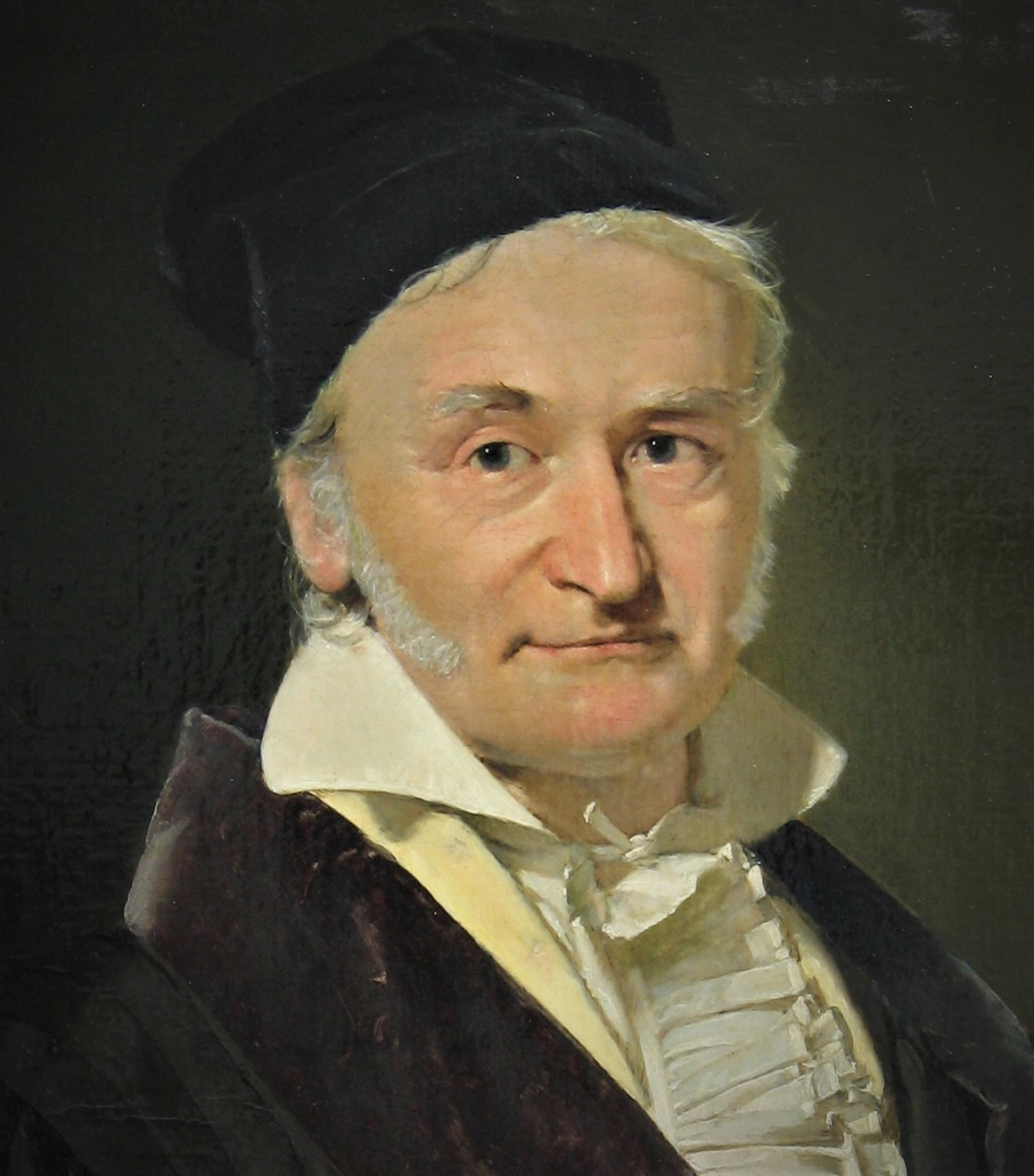Number Theory Home

## Introduction

God made the integers, all else is the work of man. --- Leopold Kronecker

Why are numbers beautiful? It's like asking why is Beethoven's Ninth Symphony beautiful. If you don't see why, someone can't tell you. I know numbers are beautiful. If they aren't beautiful, nothing is. --- Paul Erdös

The number theory is one of the oldest branches of mathematics which is mainly the study of integers. It used to be called "arithmetic" before the twentieth century. Carl Friedrich Gauss is said to have claimed that "Mathematics is the queen of the sciences and number theory is the queen of mathematics." Number theory has many applications including in cryptography.Carl Friedrich Gauss (1777 - 1855)

### Notation and Terminology

• $$\mathbb N \text{ or } \mathbb Z^+=\{1,2,3,\ldots\}$$, the set of natural numbers.
• $$\mathbb Z=\{0,\pm1,\pm2,\pm3,\ldots\}$$, the set of integers.
• $$\mathbb Q=\left\{\frac{p}{q}\mid p, q \in \mathbb Z \mbox{ and } q\neq 0\right\}$$), the set of rational numbers.
• $$\mathbb R$$, the set of real numbers.
• $$\displaystyle\sum_{i=1}^na_i=a_1+a_2+\ldots+a_n$$. E.g., $$\displaystyle\sum_{i=1}^n i=\frac{n(n+1)}{2}$$
• $$\displaystyle\prod_{i=1}^na_i=a_1a_2\cdots a_n$$. E.g., $$\displaystyle\prod_{i=1}^n i=:n!$$

A number theoretic notation:
$$d$$ divides $$n$$ (in $$\mathbb Z$$ ) means $$n=kd$$ for some $$k\in \mathbb Z$$. We denote it by $$d\vert n$$. For example, $$2\vert 8$$ and $$2\nmid 9$$.

Last edited